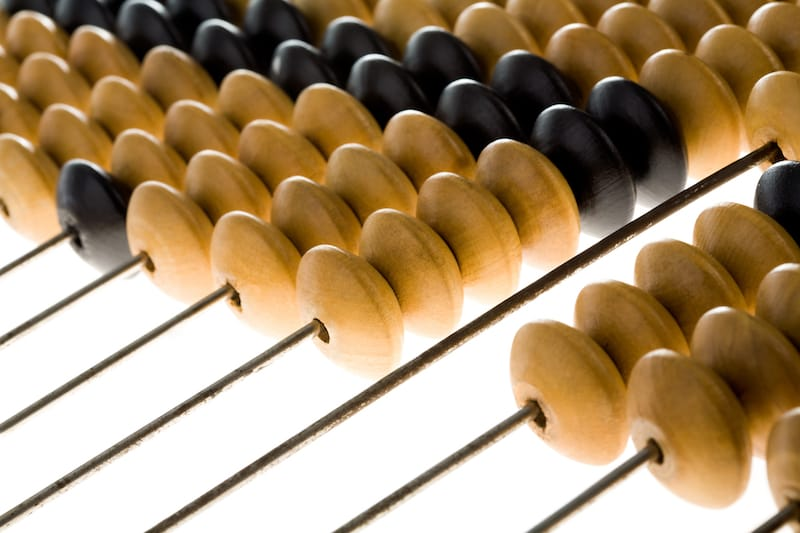Do you remember how you first learned mathematics? After you got past counting numbers, right away there was arithmetic. Math might have seemed a piece of cake until the numbers started getting bigger. To so many students this was where math anxiety started to take place. Sometimes the numbers seemed impossibly big and the memorization of simple arithmetic was not getting them to the answers.

Why Teach the Abacus

Many young adults can understand how to put together small numbers to make larger numbers or how to see large numbers as a composite of small numbers. This process is called decomposition. What parents and educators need to strive for is teaching decomposition at an early age before math anxiety has a chance to set in. Children who learn the abacus at a young age understand decomposition in the same way adults do. They solve large multi-numbered arithmetic easily because they can see, mentally and physically on the abacus, how to decompose these numbers.

What is Decompostion?

Mathematical decomposition is breaking down a large number into its smaller parts and manipulating those parts to produce the answer to the problem. For example:

4,782 + 12,936 is

4,782 + 10,000 + 2,000 + 900 + 30 + 6

First we add 10,000 to 4,782

14,782 + 2,000 + 900 + 30 + 6

14,782 + 2,000 is simple enough. 16,782

16,782 + 900 + 30 + 6

Well this next step is 782 + 900. Here it might get a little worrisome, but if I look at
a decomposition of 10 as 9 - 1, then I can see that this is 17,682.

17,682 + 30 + 6

Again 83 + 30, but remembering my decomposition I learned on the abacus I see that this is 17,712

17,712 + 6

Adding the 6 to finish it up, I have...

17,718

Now imagine doing all of that in your head in a matter of seconds. A student who learns the abacus does not have to wonder if they can or cannot add four or more digit numbers in their head. They know they can because they know the abacus. Decomposition is an invaluable tool that is a part of every mathematics’ curriculum.

Abacus and Decomposition

While they are many ways to teach decomposition, the most efficient and the longest lasting way is through the abacus. When a student learns the abacus they learn decomposition from the start. It is literally built into the abacus. Other programs may give your student colorful puzzle pieces to show how numbers “fit together”, once they have memorized numbers 1 to 20 or 1 to 100. Abacus learning is teaching decomposition as your student learns numbers, not after. In this way an abacus student doesn’t question how three and four digit numbers can be manipulated. There isn’t a worry about the numbers being “too big”. In a short amount a time a child will understand how every large number is composed and how to do any arithmetic operation with any amount of numbers.

The heart of abacus - and decomposition - learning is the “partners” or “buddies”. These are the number pairs that create 5 and 10. For example, 3 and 2 make 5, they are “little buddies”, 3 and 7 make 10, they are “big buddies” Buddies are interchangeable (-reciprocal?). So if 3 is the little buddy of 2, 2 is the little buddy of 3. This method is effective and to the point. As the child manipulates the abacus, they are physically interacting with the numbers. They see and feel what the buddies are and how they work. At the end of the day the student and parents know that the buddies are reliable and memorable way to understand number relations.

The abacus teaches pattern recognition and number relations comparable to college mathematics in a way that a three-year-old understands. It sounds too good to be true but our success stories speak for themselves. As an abacus student is solving these math problems they are always checking “what is the big buddy of ___?” “what is the little buddy of ___?”. As an abacus student is mastering decomposition, they are able to do computations such as 4782 + 12936 + 751 -873 in their head. Since an abacus student knows these number pairs they know decomposition, because of this they understand how large mathematical problems are nothing scary at all.

1

## What To Do Next…

1 Get your free 60-minute Child Assessment and learn:

• If your child is learning at the appropriate age level.